# Solving the Schrödinger equation of hydrogen molecules with the free-complement variational theory: essentially exact potential curves and vibrational levels of the ground and excited states of the Σ symmetry†

Yusaku I. Kurokawa*, Hiroyuki Nakashimaand Hiroshi Nakatsuji*
Quantum Chemistry Research Institute, The Kyoto Technoscience Center 16, 14 Yoshida Kawara-machi, Sakyo-Ku, Kyoto 606-8305, Japan. E-mail: y.kurokawa@qcri.or.jp; h.nakatsuji@qcri.or.jp

Received 21st September 2018 , Accepted 22nd October 2018

First published on 27th November 2018

The Schrödinger equation of hydrogen molecules was solved essentially exactly and systematically for calculating the potential energy curves of the electronic ground and excited states of the 1Σg, 1Σu, 3Σg, and 3Σu symmetries. The basic theory is the variational free complement theory, which is an exact general theory for solving the Schrödinger equation of atoms and molecules. The results are essentially exact with the absolute energies being correct beyond μ-hartree digits. Furthermore, all of the present wave functions satisfy correct orthogonalities and Hamiltonian-orthogonalities to each other at every nuclear distance along the potential curve, which makes systematic analyses and discussions possible among all the calculated electronic states. It is noteworthy that these conditions were not satisfied in many of the accurate calculations of H2 reported so far. Based on the present essentially exact potential curves, we calculated and analyzed the vibrational energy levels associated with all the electronic states. Among them, the excited states having double-well potentials showed some interesting features of the vibrational states. These results are worthy of future investigations in astronomical studies.

## I. Introduction

Solving the Schrödinger equation (SE) is a paramount theme of quantum chemistry because it is a governing principle of chemical phenomena. A general exact theory for solving the SE was proposed by one of the authors.1–5 It was initially called free ICI theory and later renamed as free complement (FC) theory. The FC theory produces the complement functions (cf's) {ϕi} with which the exact solution of the SE is described as ψ = ∑Ciϕi: the FC wave function has a potentially exact structure so that after applying the variational principle, we can get the exact wave function. This FC-variation principle (VP) method3 is straightforward and accurate when one can evaluate the matrix elements in the Hamiltonian and overlap matrices analytically, though such cases are limited only for some small systems. We have applied the FC-VP method to solve the SE of small systems, like helium atoms,6–8 hydrogen molecular ions in the ground and excited states in both Born–Oppenheimer (BO) and non-BO levels,9–12 and hydrogen molecules at the equilibrium geometry in the ground state.13 Their solutions were extremely accurate with satisfying the variational principle: the calculated absolute energies always keep upper bounds to the exact ones.

On the other hand, when analytical evaluations of the overlap and Hamiltonian matrices over the complement functions are difficult, we use the local SE's at many sampling points over the target atoms or molecules as the conditions to determine the coefficients of the complement functions. This method is called the local Schrödinger equation (LSE) method.4 The LSE method is an integral-free method, so that one can apply it to any systems and any type of cf's. This method has been applied to various atoms and small organic and inorganic molecules and gave highly accurate energies satisfying chemical accuracy (less than 1 kcal mol−1 as the absolute energy) for both the ground and excited states.5,14–17

In this study, we systematically investigate the electronic ground and excited states of the hydrogen molecule. We solve the BO SE of a hydrogen molecule in the ground and lower excited states almost exactly by the FC-VP theory: all the integrals involved are calculated analytically. We calculate highly accurate PECs of the 1Σg, 1Σu, 3Σg, and 3Σu symmetries. The calculated wave functions at each nuclear distance R of state n, ψn(R), satisfy

 Ĥ(R)ψn(R) = En(R)ψn(R), (1)
 〈ψn(R)|ψm(R)〉 = δmn, (2)
and
 〈ψn(R)|Ĥ(R)|ψm(R)〉 = En(R)δmn, (3)
where En is the electronic energy of state n. Then, from these PECs, we systematically calculate almost all the vibrational energy levels associated with each electronic state.

The study of the accurate wave functions of hydrogen molecules has a long history since it is the simplest molecule and is very often used as the benchmark study for new quantum chemistry calculations. In 1933, as a pioneering study, James and Coolidge (JC)18 succeeded in obtaining very accurate energies and the equilibrium geometries of the hydrogen molecule. Sims and Hagstrom improved the JC type wave function and obtained the PECs in the 1Σg symmetry.19 In 1965, Kolos and Wolniewicz (KW) reported the wave functions20 that cover the whole region of the internuclear distance R for the ground and several excited states. The KW type wave functions were applied to various states of different spin and spatial symmetries,21–29 but their methods were state-specific so that their wave functions were not guaranteed to be orthogonal and Hamiltonian-orthogonal with those in the other states. In 1995, Cencek, Komasa, and Rychlewski showed that the explicitly correlated Gaussian (ECG) type wave functions give very accurate energies for the 3Σg, 3Σu, 1Σu, 1Πu, and 1Σg states.30 Adamowicz et al. also performed non-adiabatic calculations using the ECG wave functions and very accurate vibrational levels for the ground state.31 In 2009, Corongiu and Clementi employed the full CI method and calculated the PECs of the ground and 12 excited states in the 1Σg state.32,33 Recently, in 2013, Pachucki reported very precise energies for the 1Σg states at some R values.34,35 The accurate calculations of the triplet states: 3Σg and 3Σu symmetries were also reported by Hagstrom et al. and Pachucki and Komasa.36,37 Also, the PECs of several states were reported by the experiments.38,39

In most of the above literature of the theoretical studies, several target states were selected and computed state-specifically. Therefore, the physical features among the states had not been discussed systematically. On the other hand, in the present study, all the calculated states satisfy orthogonality and Hamiltonian orthogonality to each other between the different states, which is a necessary condition for the exact solutions of the Schrödinger equation. This is so for all the distances along the potential energy curves, from which we systematically calculated the vibrational energy levels associated with each potential curve of a different state.

In the present FC calculations, we dealt with the λ functions with negative powers which were used originally in the previous study of hydrogen molecules with the FC theory.13 This type of functions (defined in Section IIB) is very important for small R and near-equilibrium distance,13 but these λ functions have not used in other literature studies.

Recently, we were also successful in calculating the highly accurate PECs of the ground and various excited states by the FC-LSE method, i.e. using a sampling method. With the FC-LSE method, the computations are much easier than the present analytical FC-VP method since no integrations are necessary there, though the variational principle is not always satisfied and special care about sampling dependency is required. The results of the FC-LSE calculations will be published elsewhere.40

## II. Free complement theory

The FC theory is a general theory of solving the Schrödinger equations, and the details of this theory were explained in previous studies.2,3,5 We explain here only the points pertinent to the present study. The FC wave function of order n is represented as a linear combination of the complement functions (cf's) {ϕ(n)}, given by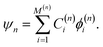(4)
The coefficients {C(n)} are determined by the variational principle, i.e., by solving the secular equation, HC = ESC, where H and S are the Hamiltonian and overlap matrices, respectively. Each energy value is the upper bound to the exact energy according to the variational principle, and the wave function is always orthogonal to each other since both H and S are Hermite matrices. The {ϕ(n)} is generated from an initial function ψ0 by using the following simplest iterative complement (SIC) formula,
 ψn+1 = [1 + Cng(Ĥ − En)]ψn, (5)
where g is a scaling function that was introduced to overcome the singularity problem caused by the singular Coulomb potentials existing in the Hamiltonian of atoms and molecules.2,3,5Cn and En are the coefficient and energy at the iteration n in the SIC formula, respectively. We perform the operation of eqn (5)n times from ψ0 and select only linearly independent and non-divergent functions as {ϕ(n)} in the right-hand side of eqn (5). The number of the selected function is defined as dimension: M(n). The ψn determined by eqn (4) is guaranteed to converge to the exact solution of the SE as the order n increases.2,3,5

### A. Initial wave function

In the FC theory, we first need to select an appropriate initial wave function ψ0. The converging speed to the exact solution depends on the choice of ψ0. In our previous study of hydrogen molecules for the ground state,13 we employed the JC type function as ψ0. In the present study, we modified it and employed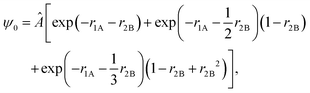(6)
as our initial wave function, where riX represents the distance between electron i and nucleus X (X = A or B), and Â is the symmetry-adaptation operator, which will be explained in Section IIC. The first, second, and third terms in the brackets in eqn (6) ensured that the wave function correctly dissociates to H(1s)–H(1s), H(1s)–H(n = 2), and H(1s)–H(n = 3) states, respectively. We fixed the orbital exponents (the coefficient of riX in the exp functions) as given in eqn (6) in the whole regions of the PECs. This has three merits: the first is that the resultant PECs dissociate into the correct states, the second is that the PECs are smooth because we do not do any non-linear optimizations at each R, and the third is that one can save the computational time. The demerit of the fixed exponents is that the descriptions of the wave function in the bonding region may become worse than those of the dissociated region. This demerit, however, can be overcome with the FC theory automatically by increasing its order.

### B. Hamiltonian and complement functions in elliptic coordinates

We employ the elliptic coordinates whose variables are defined as λi ≡ (riA + riB)/R, μi ≡ (riAriB)/R, where R is the distance between the nuclei A and B, and φi, which is the azimuthal angle around the molecular axis (i = 1 and 2). We further use supplementary variables, ρ, defined by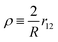.

Using the elliptic coordinates, the initial wave function (6) is rewritten generally as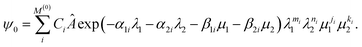(7)
The exponents α and β in eqn (7) change as the inter-nuclear distance changes due to the existing R in λ and μ coordinates.

The kinetic operator and the potential in the Hamiltonian are written as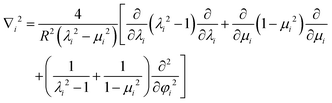(8)
and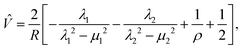(9)
respectively, in the elliptic coordinates. The g function employed in this study is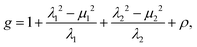(10)
where the second and third terms correspond to the inverse orders of the electron–nucleus potentials of electrons 1 and 2, 1/r1A + 1/r1B and 1/r2A + 1/r2B, respectively, and the fourth term corresponds to the inverse order of the electron–electron repulsive potential 1/r12. When we operate the kinetic and potential operators and the g function on ψ0 according to eqn (5), we obtain the general expression of the cf's as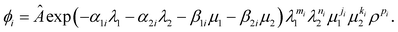(11)
Note that the integers j, k, and p that appear as the power of μ1, μ2, and ρ, respectively, take zero and positive and the integers m and n (the power of λ1 and λ2, respectively) take negative, zero, and positive in the cf's. The cf's with the negative power of λ1 and/or λ2, which have not been used in the other literature studies, are our original and first used in our study. Their efficiencies were examined in the previous study,13 and they would be important to describe especially the bonding region.

### C. Symmetry adaptation

The symmetry adaptation operator, Â, in eqn (6) is defined as Â =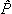spinspace, where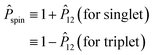(12)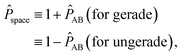(13)
and12 interchanges the electrons 1 and 2 as12 = {λ1λ2, μ1μ2} andAB interchanges the nuclei A and B asAB = {μ1 ↔ −μ1, μ2 ↔ −μ2}. The combinatorial use of these operators can express the 1Σg, 1Σu, 3Σg, and 3Σu states from the common initial wave function.

### D. Integral evaluation

The Hamiltonian matrix H and the overlap matrix S of the cf's are defined by Hij ≡ 〈ϕi|Ĥ|ϕj〉 and Sij ≡ 〈ϕi|ϕj〉, respectively. The integrands are the linear combination of the following basic integrals: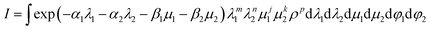(14)
where j, k, and p take zero or positive integers, m and n take negative, zero, or positive integers, the integration ranges of each of the variables are, 1 ≤ λi ≤ ∞, −1 ≤ μi ≤ 1, and 0 ≤ φi ≤ 2π(i = 1, 2), and the Jacobian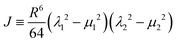is already considered and expanded in the integrands.

To evaluate eqn (14), the ρp is reduced to ρ0 when p is even or to ρ−1 when p is odd using the relation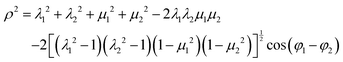(15)
repeatedly.

The ρ−1 can be expanded using the Neuman's expansion,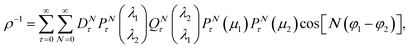(16)
where D0τ = 2τ + 1, DNτ = 2(2τ + 1)[(τN)!/(τ + N)!]2 (N > 0), and P and Q are the associated Legendre functions of the first and second kinds, respectively, and we take the upper variables when λ2λ1 and the lower ones otherwise. Then, the integrand in eqn (14) is decomposed into a sum of products of λ1 and λ2 parts, the μ1 part, the μ2 part, and the φ1 and φ2 parts. We can integrate over these variables separately. In the τ summation in eqn (16), we truncate it when the summed value reaches in more than 60-digit accuracy. In integrating the λ2 part, we need to evaluate the integral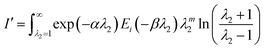(17)
and related ones. These integrations including the Ei functions with negative m were calculated numerically using the Maple program.41 All the other calculations were done using the GMP (GNU multiple precision arithmetic) library.42

## III. Convergence of the FC wave function

In Table 1, the numbers of the cf's M(n) generated at order n = 0, 1, 2, and 3 are presented for each symmetry, 1Σg, 1Σu, 3Σg, and 3Σu. A set of the cf's at order n always includes those at lower orders m (m < n). At order n = 0, the number of the initial wave functions is M(0) = 10 for the 1Σg and 3Σu symmetries, but M(0) = 9 for the 1Σu and 3Σg symmetries. This is because the exp(−r1Ar2B) term in the initial wave function, which represents the H(1s)–H(1s) state at the dissociation limit, vanishes when the symmetry-adaptation operator is applied as explained in Section IID. For the same reason, the numbers of the generated cf's are different for each symmetry at order = 1, 2, and 3. All cf's at order n = 3 in the 1Σg, 1Σu, 3Σg, and 3Σu states are listed in Tables S1–S4, respectively, in the ESI.
Table 1 Number of the complement functions (cf's) at order n = 0, 1, 2, and 3 for different symmetries
State Order
0 1 2 3
1Σg 10 63 422 1819
1Σu 9 58 386 1642
3Σg 9 58 388 1609
3Σu 10 63 424 1786

We calculated the energies and the FC wave functions, changing the inter-nuclear distance R at order n = 0, 1, 2, and 3. We call the lowest eigenvalue in each symmetry E0, and second, third,… solutions E1, E2,…. We also use the Herzberg's notation for known states. To check the convergence of the FC wave functions, Fig. 1 shows the PECs of the 1Σg state at n = 0, 1, 2, and 3, and their energies are shown in Table 2 at the selected inter-nuclear distances. As the order n increases, the energies converge from the above to the exact values cited at the bottom of each distance in Table 2. It was observed that, at n = 2, the energies of each state have more than 3-digit accuracies at each distance, except for short inter-nuclear distances in the E2(GK), E3(H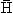), E4(P) and E5(O) states. At n = 3, the energies of each state almost converged to the exact values, and they have μ-hartree accuracies or better, as discussed below. These observations hold true for the other symmetry states. In the following discussions, we use the current best results at n = 3, if not specially mentioned.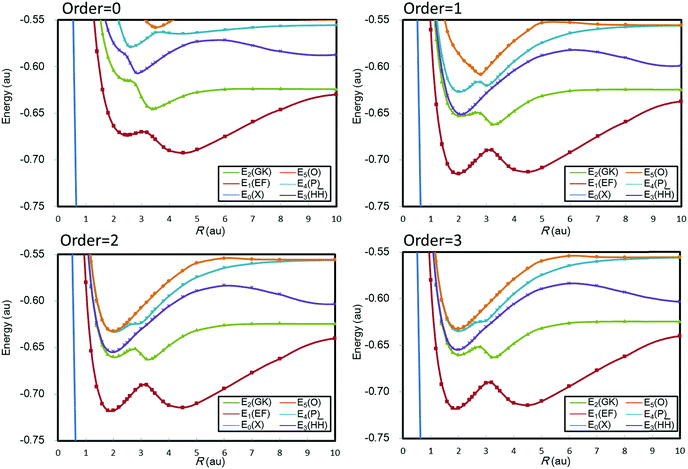Fig. 1 Convergence of the FC energies at order n = 0, 1, 2, and 3 for the potential energy curves of hydrogen molecules in the 1Σg state.
Table 2 The energies (a.u.) of hydrogen molecules at the selected inter-nuclear distances in the 1Σg state calculated with the FC method at order n = 0, 1, 2, and 3a
R (a.u.) Order E 0(X) E 1(EF) E 2(GK) E 3(H) E 4(P) E 5(O)
a The complete data at order n = 3 for the 1Σg, 1Σu, 3Σg, and 3Σu symmetries are listed in Tables S5–S8 in the ESI. b c d e
0.5 0 −0.473160090 0.467150166 0.526489788 0.554210586 0.647965456 0.748789199
1 −0.522270516 0.178662300 0.271787939 0.302046504 0.307437497 0.362205778
2 −0.526517488 0.127170779 0.206447033 0.210128548 0.233044870 0.238357218
3 −0.526636133 0.127042609 0.205960702 0.209276728 0.232336865 0.233707889
Ref. −0.526638758b 0.127044530c

1.0 0 −1.097460370 −0.400906655 −0.340543336 −0.312559747 −0.219768603 −0.176895708
1 −1.122793990 −0.560602662 −0.487470814 −0.467911114 −0.459860854 −0.401596139
2 −1.124496291 −0.580068223 −0.508024388 −0.507783883 −0.483221875 −0.479786999
3 −1.124538673 −0.580085427 −0.508066032 −0.507859752 −0.483310834 −0.483260350
Ref. −1.124539720b −0.580085101c −0.508065352d −0.507857287d −0.483309219d −0.483247664d

1.4011 0 −1.151637266 −0.583738232 −0.523262560 −0.495015865 −0.409139152 −0.387201495
1 −1.173265053 −0.683500301 −0.616199031 −0.604979087 −0.589610053 −0.536101526
2 −1.174450788 −0.692155449 −0.626657136 −0.624549899 −0.600828388 −0.599117068
3 −1.174475307 −0.692162609 −0.626679488 −0.624559939 −0.601824405 −0.600880012
Ref. −1.174475931b

2.0 0 −1.119487252 −0.663841484 −0.604125928 −0.575030024 −0.531480597 −0.464243660
1 −1.137224888 −0.714988763 −0.652681227 −0.650634303 −0.626984693 −0.586189922
2 −1.138116836 −0.717711177 −0.660423055 −0.654923977 −0.633261515 −0.632402335
3 −1.138132582 −0.717715211 −0.660430062 −0.654926430 −0.634909096 −0.632458511
Ref. −1.138132957b −0.717714276d −0.660428175d −0.654926063d −0.634885512d −0.632457557d

3.0 0 −1.047489133 −0.670000092 −0.632345999 −0.605622343 −0.573958494 −0.544594688
1 −1.056767266 −0.690228452 −0.656010205 −0.628286094 −0.620220068 −0.602579339
2 −1.057316865 −0.690744333 −0.656978883 −0.630548949 −0.623906192 −0.607306364
3 −1.057326071 −0.690747023 −0.656985802 −0.630554115 −0.623922686 −0.607983717
Ref. −1.057326269b −0.690747056e −0.656985945e −0.630554134e −0.623922735e −0.607984513e

6.0 0 −1.000100834 −0.659400228 −0.623820422 −0.577252084 −0.558001450 −0.547795548
1 −1.000815298 −0.692111313 −0.625995288 −0.582171944 −0.564240910 −0.552563424
2 −1.000835357 −0.694242391 −0.626146298 −0.583447929 −0.564423020 −0.553887236
3 −1.000835702 −0.694266430 −0.626147956 −0.583463397 −0.564424570 −0.553905248
Ref. −1.000835708b −0.694267029d −0.626147969d −0.583463574d −0.564424574d −0.553905272d

10.0 0 −1.000000522 −0.629934919 −0.624203739 −0.587207122 −0.555495453 −0.549842549
1 −1.000008459 −0.637305568 −0.624627378 −0.599068694 −0.555923767 −0.555457695
2 −1.000008753 −0.640213070 −0.624640969 −0.603362241 −0.555999881 −0.555538697
3 −1.000008756 −0.640253722 −0.624641133 −0.603416613 −0.556000843 −0.555539183
Ref. −1.000008756b −0.640246025d −0.624641106d −0.603407271d −0.556000540d −0.555538599d

## IV. Potential energy curves

In Fig. 2, all the ground and excited PECs in the 1Σg, 1Σu, 3Σg, and 3Σu symmetries and vibrational levels associated with each PEC are shown. In Fig. 3–6, the PECs of the lowest six states of the Σ symmetry are separately plotted. The detailed energy values for each R are given in Tables S5–S8 of the ESI. Note that the sixth states of the 1Σu and 3Σg symmetries, which dissociate to the H(1s)–H(n = 4) states, are not guaranteed to dissociate to the correct states in the present method because the proper wave functions for the H(1s)–H(n = 4) states are not included in the initial wave function. In the FC theory, however, the higher order of the cf's from other initial functions can accurately cover these states. We again emphasize that the present calculations are not state-specific: the ground and excited states are common eigenfunctions of the same Hamiltonian matrix of each symmetry, 1Σg, 1Σu, 3Σg, and 3Σu, at each R. This makes systematic discussions possible for all the calculated states and their PECs.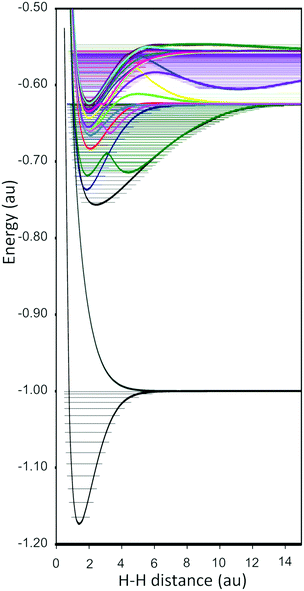Fig. 2 All ground and excited potential energy curves (PECs) and vibrational levels associated with each PEC.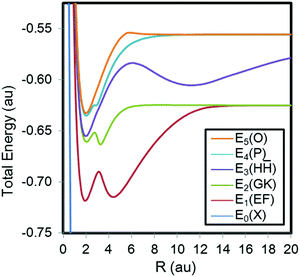Fig. 3 The 1Σg potential energy curves of hydrogen molecules calculated by the FC method at order n = 3.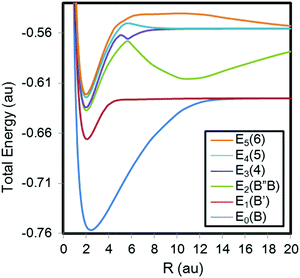Fig. 4 The 1Σu potential energy curves of hydrogen molecules calculated by the FC method at order n = 3.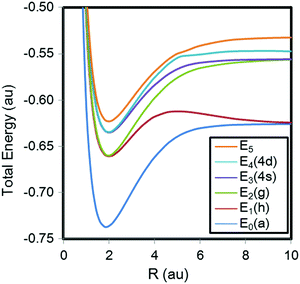Fig. 5 The 3Σg potential energy curves of hydrogen molecules calculated by the FC method at order n = 3.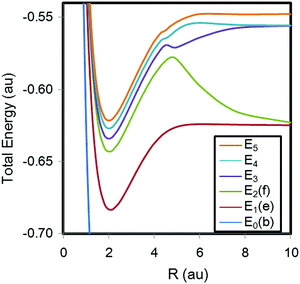Fig. 6 The 3Σu potential energy curves of hydrogen molecules calculated by the FC method at order n = 3.

For the ground state of the 1Σg state, the total energy E0(X) at the equilibrium geometry (R = 1.4011 a.u.) is calculated to be E = −1.174475307 a.u. as seen from Table 2. This has a μ-hartree accuracy compared with the reference energy: E = −1.174475931400216 a.u. from the 22363-basis calculations by Pachucki.34 The energies at R > 1.4 a.u. also have μ-hartree accuracies and those at R < 1.4 a.u. have 5-digit accuracies, compared with those by Pachucki.34

The excited states of the 1Σg symmetry are also calculated accurately compared with the best values in the literature studies; the differences between the FC energies and the Pachucki's energies35 are calculated to be ΔE0 = 1.98 × 10−7 a.u., ΔE1 = 3.30 × 10−8 a.u., ΔE2 = 1.43 × 10−7 a.u., ΔE3 = 1.90 × 10−8 a.u., ΔE4 = 4.90 × 10−8 a.u., and ΔE5 = 7.96 × 10−7 a.u. for the first (EF), second (GK), third (H), fourth (P), and fifth (O) excited states, respectively, at R = 3.0 a.u. At R = 6.0 a.u., the energies are calculated to more than 7-digit accuracies. Similarly, the 1Σu,283Σg,27,36 and 3Σu27,36,37 states are calculated to more than 5-digit accuracy in the bonding regions (R = 2.0 a.u.).

It was observed that the FC energy becomes more accurate with increasing R. For example, the FC energy has more than 10-digit accuracy at R = 10.0 a.u. in the 1Σg state (the FC energy is E0 = −1.000008755715 a.u., and Pachucki gave E0 = −1.000008755746 a.u.),34 while the FC energy has a μ-hartree accuracy at the equilibrium geometry, as mentioned above. This observation applies also to the excited states. The reason why the FC energies become more accurate with increasing R is that the initial wave functions of the FC wave functions were chosen to be exact at the completely dissociated geometry, as explained in Section IIA.

At short inter-nuclear distances (R < 1.0 a.u.), where the potential curve is repulsive and steep, the energies are less accurate; for example, the energy of the E4 state in the 1Σu symmetry at R = 1.0 a.u. has a milli-hartree accuracy.28 This is because we do not include an appropriate wave function as the initial function for short R. To study very short inter-nuclear regions, it may be better to include a function for a united atom, such as the Chemical Orbital proposed by Clementi and Corongiu.33

We also note that, at many points of the calculated PECs, our results gave better energies than ever reported values; for example, our FC theory gave the energy E5 = −0.632458510 a.u. at R = 2.0 a.u. of the 1Σg state, while Wolniewicz and Dressler gave E5 = −0.632457557 a.u.25 But at some points, our energies are a little worse than the reported values; for example, in the ground state of the 1Σg symmetry, Pachucki gave better energies in the whole region.34 However, it seems that our values have more than 5-digit accuracies in the bonding and dissociation regions of the PECs of the 1Σg34 and 1Σu,28 3Σg,27,36 and 3Σu27,36,37 states. However, note that we have used less than 2000 functions to describe simultaneously the ground and excited states of each symmetry (see Table 1), whereas Wolniewicz and Dressler used the different basis function sets for different states since their calculations were state-specific.

It is known that the potential energy curves of some excited states have van der Waals minima at large nuclear distances:29 Staszewska et al. gave the minima in the b3Σu state (the ground state in the 3Σu state) at R = 8.0 a.u. with 4.4369 cm−1 depth and in the e3Σu state (the first excited state in the 3Σu state) at R = 15.0 a.u. with 2.9993 cm−1 depth (the depth is the difference between the energy of the dissociation limit and the local minimum energy).27 The present FC theory gives the minima for the b3Σu state at R = 8.0 a.u. with 4.4380 cm−1 depth and in the e3Σu state at R = 15.0 a.u. with 2.9998 cm−1 depth (see Table S8, ESI). They gave another minimum in the h3Σg state (the first excited state in the 3Σg state) at R = 15.6 a.u. with 1.8338 cm−1 depth,27 while the FC theory gives it at R = 15.6 a.u. with 1.8339 cm−1 depth (see Table S7, ESI). Thus, the present method can describe such a small van der Waals interaction very accurately.

In Fig. 7, we also show the potential energy curves of the excited states higher than the sixth state for the 1Σg symmetry, and their detailed values are listed in Table S9 (ESI). Corongiu and Clementi already reported 12 excited states by the full CI method,32 but we report here the 15 states by the FC-VP method. These energies are obtained as the higher eigenvalues of the secular equation and are the upper bounds to the exact values, but there are no initial functions corresponding to their dissociations. Nevertheless, these data would be still accurate and become useful references for the future theoretical and experimental studies.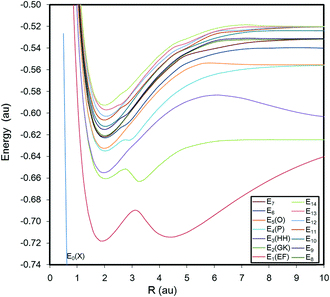Fig. 7 The 1Σg potential energy curves in the higher excited states of hydrogen molecules calculated by the FC method at order n = 3.

Fig. 8 and Fig. S1 (ESI) summarize all the PECs of the 1Σg, 1Σu, 3Σg, and 3Σu symmetries, calculated in this paper. The lowest-energy curves of 1Σg and 3Σu dissociate to H(1s) + H(1s), but there are no such dissociation channels in 1Σu and 3Σg. This is trivially understandable from their spatial and spin symmetries. By comparing the PECs of 1Σg and 1Σu with those of 3Σg and 3Σu, the former includes more complicated curves. This is due to the existence of the ionic channels that dissociates to H + H+ in 1Σg and 1Σu. On the other hand, no ionic channels exist in 3Σg and 3Σu where two electrons cannot occupy the same position in H due to the Pauli repulsion. At the dissociation limits of 1Σg and 1Σu, the energy of the ionic state: H + H+ is higher than that of H(1s) + H(n = 3). However, since the ionic contribution indicates a long-range interaction proportional to 1/R, the ionic curve moves from lower states to higher states, step by step, as approaching dissociation. Thus, the existence of the ionic term makes the PECs complicated and causes double-well potential shapes and state repulsions. Although the ionic channels indicate long-range behavior, the PECs showing that the ionic characters are dominant almost overlap between 1Σg and 1Σu at large R, for example, compare the EF state of 1Σg with the B state of 1Σu. This indicates that the origin of the long-range behavior is mainly explained from the classical ionic Coulombic interactions. In the present calculations, we do not explicitly employ the ionic terms, such as exp(−r1Ar2A), into our initial functions. Nevertheless, all the PECs including ionic contribution can be accurately described at least near equilibrium distances. This is a numerical proof that the FC wave function converges to the exact solutions with increasing the FC order from any initial functions at the same symmetry. Actually, the cf's including λm terms, where m runs positive and negative integers, are two-centered functions and contain the ionic characters also with the covalent characters. However, explicitly introducing ionic-type functions into the initial function, the excited states containing ionic characters can be more efficiently described, especially for near the dissociations and/or higher excited states. In the forthcoming paper40 by the FC-LSE method, we employ such ionic terms explicitly as the initial function and will do further discussions for their states.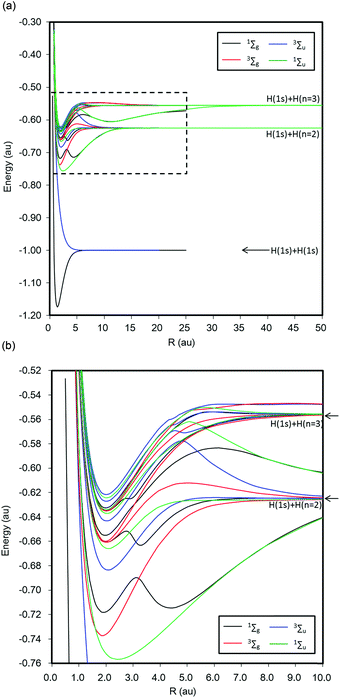Fig. 8 (a) Potential curves of all the Σ states calculated by the FC method at order n = 3. The black, blue, red, and green curves are for the 1Σg, 3Σu, 3Σg, and 1Σu symmetries, respectively. The region in the broken line is enlarged in (b). (b) Enlarged figure of (a). Potential curves of all the Σ states calculated by the FC method at order n = 3. The black, blue, red, and green curves are for the 1Σg, 3Σu, 3Σg, and 1Σu symmetries, respectively.

## V. Vibrational energy levels

Thus, we could evaluate very accurate PECs for the ground and excited states of Σ symmetries by the FC-VP method. Since their PECs are essentially exact, it is valuable to proceed to the vibrational analysis based on their accurate PECs. Here, we numerically solve the vibrational Schrödinger equations, given by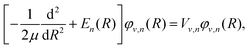(18)
where En(R) is a potential energy function obtained by fitting the discrete PEC of state n with appropriate fitting functions, μ is the reduced mass, and φv,n(R) and Vv,n are the vibrational wave function and the corresponding energy of the v-th state, respectively. For solving eqn (18), we actually used the Level 8 package by Le Roy.43 Since the number of the points of R that we calculated at n = 3 is limited, the highly accurate discrete PECs were interpolated and extrapolated and then obtained En(R): for the interpolation, the cubic spline method was employed, and for the extrapolation of the outer region of the PECs, the energy values were fitted to the function E(R) = EA/RB, and for the extrapolation of the inner region of the PECs, the energy values were fitted to the function E(R) = E0 + Aexp(−BR).43 Then, the vibrational SEs for each PEC in the 1Σg, 1Σu, 3Σg, and 3Σu symmetries were solved numerically, and the vibrational energy levels Ev, the expectation value of the nuclear distances 〈R〉, and those of the squared values 〈R2〉 were obtained associated with all the calculated potential energy curves.

In Fig. 9, the calculated vibrational levels associated with the PECs of the ground and excited states are plotted. The vibrational levels in the sixth states of the 1Σu and 3Σg symmetries, which dissociate to the H(1s)–H(n = 4) states, are not calculated since the PECs do not dissociate correctly, as explained in Section IV. The complete data for all the calculated vibrational levels are listed in Tables S10–S13 in the ESI, where we listed only the lowest 50 vibrational states for the E3(H) 1Σg and E2(B′′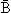) 1Σu states because very high energy states would be highly dependent on the fitting method and may be unphysical. The ground state of the 3Σu curve has no vibrational state because it is a repulsive curve. The three, six, one, and one highest vibrational levels for the E1(h)3Σg, E2(f)3Σu, E4(5)1Σu, and E5(O)1Σg curves, respectively, are unstable because the outer region of these curves are repulsive and these vibrational levels are higher than the dissociation limit of their curves in energy. Fig. 10–13 are the calculated vibrational levels associated with the 1Σg, 1Σu, 3Σg, and 3Σu PECs, respectively. It is observed that, in the 1Σg and 1Σu states, the densities of the vibrational levels are sparse between E = −0.625 and −0.604 a.u. This is due to the very broad wells of the E3(H) 1Σg and E2(B′′) 1Σu curves.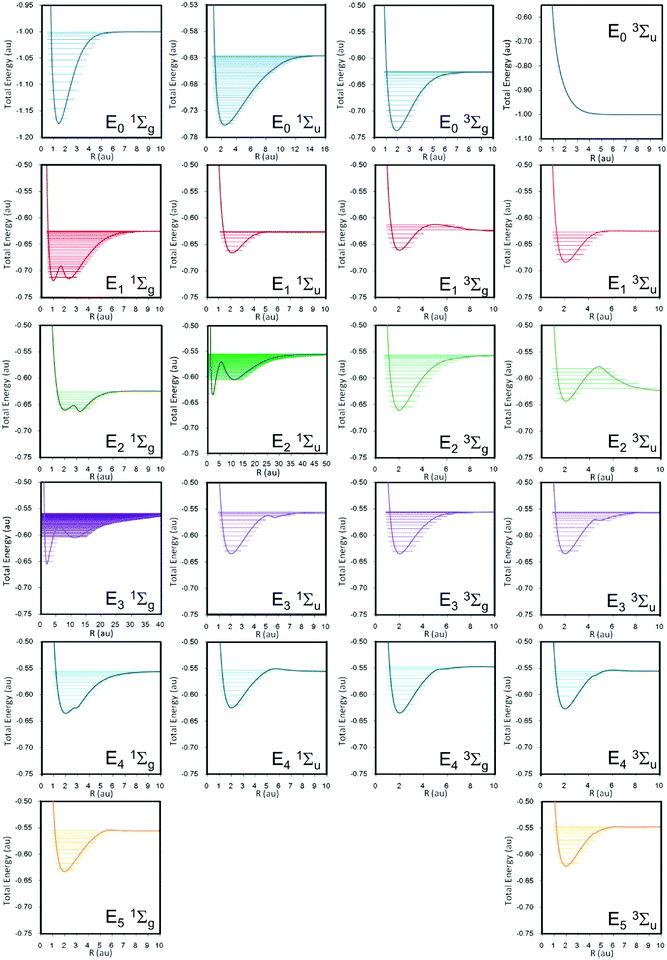Fig. 9 All vibrational energy levels associated with each PEC calculated by the FC method at order n = 3. The numerical data are listed in Tables S10–S13 of the ESI.†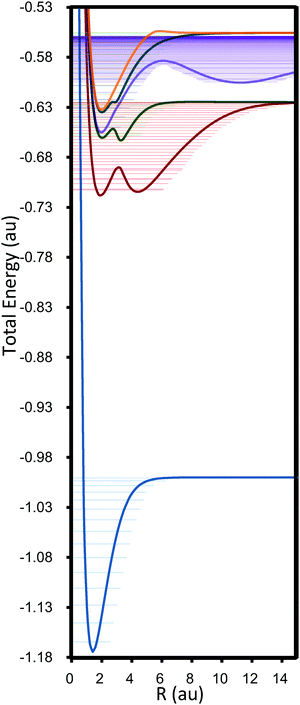Fig. 10 All vibrational energy levels associated with the 1Σg PECs. The detailed energy values are given in Table S10 of the ESI.†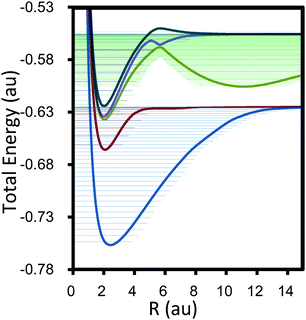Fig. 11 All vibrational energy levels associated with the 1Σu PECs. The detailed energy values are given in Table S11 of the ESI.†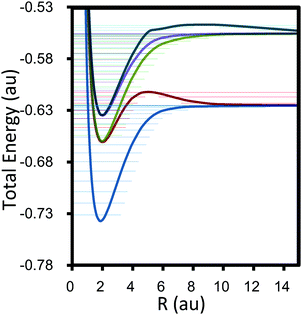Fig. 12 All vibrational energy levels associated with the 3Σg PECs. The detailed energy values are given in Table S12 of the ESI.†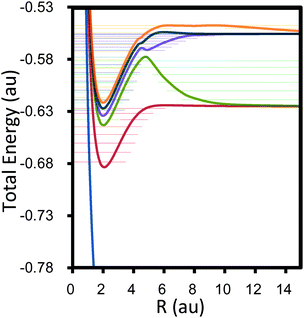Fig. 13 All vibrational energy levels associated with the 3Σu PECs. The detailed energy values are given in Table S13 of the ESI.†

Table 3 summarizes some selected vibrational energy levels of the ground (X) and three excited states (EF, GK, and H) in the 1Σg symmetry and the 〈R〉 and 〈R2〉 values, the expectation value of the nuclear distance. We compared the vibrational energy splitting: ΔEv (= EvEv−1) by the present method with those by theoretical and experimental references.22,31,39,45 For simple single-well PECs, such as the ground state (X) of 1Σg, and B state of 1Σu, the present results agreed well with those by the experiment in 2- or 3-digits. In these curves, as the vibrational level increases, the 〈R〉 and 〈R2〉 values gradually increase and the ΔEv value gradually decreases, which shows the anharmonicity of the potential. For the double-well PECs, such as the EF, GK, and Hstates of 1Σg, our results only agreed with those of the experiment within 1- or 2-digits. This would be because non-adiabatic effects become important. Wolniewicz and Dressler25 considered the non-adiabatic effects for some states and their correspondence with the experiments are improved. We do not consider their effects in the present study since the present purpose is to compute the vibrational energy levels systematically for all the calculated ground and excited states at the nonrelativistic (essentially exact) limits within the BO approximation.

Table 3 Vibrational energy levels Ev (cm−1) of the ground (X) and three low-lying excited states (EF, GK, and H) of 1Σg, calculated from the PECs of the FC method at order n = 3 and the comparisons with other studiesa
State v Assignmentb FC method Ref. (theoretical) Ref. (experimental)
Rc R2c E v d E vEv−1 E vEv−1 E vEv−1
a The complete data for all the calculated vibrational levels associated with all the PECs of the ground and excited states of the 1Σg, 1Σu, 3Σg, and 3Σu symmetries are listed in Tables S10–S13 of the ESI. b Assignments of the vibrational states for the double-well potentials. c The expectation value of the nuclear distance R in Å and that of the squared value. d Absolute energies in cm−1. e f g h
X 1Σg 0   0.76646 0.59534 −255590.81   Stanke et al.e Pardof
1   0.81797 0.69308 −251422.77 4168.03 4161.19 4161.14
2   0.87099 0.79990 −247491.76 3931.02 3925.86 3925.79
3   0.92594 0.91722 −243799.75 3692.01 3695.41 3695.43
4   0.98420 1.04843 −240340.90 3458.84 3467.99 3467.95
5   1.04705 1.19745 −237108.37 3232.53 3241.58 3241.61
6   1.11551 1.36852 −234100.24 3008.13 3013.86 3013.86
7   1.19085 1.56737 −231320.39 2779.85 2782.15 2782.13
8   1.27536 1.80350 −228777.18 2543.21 2543.19 2543.25
9   1.37269 2.09225 −226482.67 2294.51 2292.96 2292.93
10   1.48885 2.45993 −224453.96 2028.71 2026.36 2026.38

EF 1Σg 0 Left 1.04654 1.10931 −156380.80   Wolniewicz et al.g Bailly et al.h
1 Right 2.35303 5.56457 −156203.48 177.32 198.73 199.10
2 Right 2.41294 5.90780 −155009.06 1194.42 1195.67 1194.96
3 Left 1.12820 1.32172 −154053.51 955.55 936.93 935.89
4 Right 2.46532 6.23244 −153871.06 182.45 204.61 204.21
5 Right 2.50233 6.49463 −152799.62 1071.43 1081.44 1079.28
6 Left 1.51400 2.67541 −152027.49 772.13 783.97 781.37
7 Right 2.30528 5.89949 −151725.39 302.10 285.74 278.97
8   2.40554 6.35675 −150866.64 858.75 897.08 892.03
9   2.12272 5.30197 −150192.15 674.49 671.55 654.32
10   2.32806 6.30229 −149571.13 621.02 583.17 581.24
11   2.48828 7.09208 −148837.58 733.55 749.94 746.89
12   2.51076 7.31799 −148114.76 722.82 725.33 712.80
13   2.57944 7.76638 −147417.63 697.12 681.99 672.64
14   2.69065 8.41895 −146718.82 698.82 694.55 695.03
15   2.78398 9.00918 −146021.78 697.04 701.09 700.35

GK 1Σg 0 Right 1.717483 2.98119 −144365.95   Wolniewicz et al.g Bailly et al.h
1 Left 1.163475 1.400071 −143909.19 456.76 360.60 183.82
2   1.588394 2.652501 −142527.50 1381.69 1452.23 1580.81
3   1.506582 2.476543 −141689.39 838.11 764.63 651.13
4   1.707744 3.165721 −140546.42 1142.96 1145.83 1055.26
5   1.775294 3.482897 −139520.59 1025.84 1001.21 1064.81
6   1.971575 4.292311 −138589.65 930.94 892.81 916.76
7   2.237863 5.509267 −137794.47 795.17 775.20
8   2.757091 8.250715 −137235.43 559.04 523.71

H1Σg 0 Left 1.077938 1.176718 −142576.61   Wolniewicz et al.g Bailly et al.h
1 Left 1.155198 1.380249 −140358.93 2217.68 2248.49 2293.94
2 Left 1.253108 1.651700 −138350.21 2008.72 2130.59 2045.57
3 Left 1.362133 1.975955 −136601.32 1748.89 1817.18
4 Left 1.444969 2.250600 −134989.12 1612.20 1476.75
5 Left 1.545178 2.594699 −133467.32 1521.79 1556.35
6 Right 5.949931 35.496886 −132669.76 797.56 694.39
7 Right 5.980785 36.060560 −132318.58 351.18 351.03
8 Left 1.653023 2.990029 −132071.04 247.54 308.81
9 Right 6.020838 36.747809 −131977.19 93.85 32.60
10 Right 6.069392 37.552327 −131646.03 331.16 331.93
11 Right 6.120844 38.407051 −131324.35 321.68 322.63
12 Right 6.175278 39.313906 −131011.38 312.97 313.51
13 Left 1.781358 3.486536 −130804.85 206.52 262.16
14 Right 6.233125 40.280642 −130706.79 98.06 42.45
15 Right 6.294557 41.312137 −130410.71 296.08 295.83
16 Right 6.359092 42.404713 −130123.20 287.50 287.20
17 Right 6.425669 43.546667 −129844.33 278.88 278.75
18 Left 1.935205 4.123028 −129682.18 162.15 218.56
19 Right 6.491295 44.701125 −129573.90 108.27 51.81
20 Right 6.553864 45.843374 −129311.76 262.14 262.10
21 Right 6.610493 46.940327 −129057.95 253.81 253.75
22 Right 6.653100 47.902398 −128812.82 245.13 245.14
23 Left 2.154131 5.135558 −128728.19 84.63 128.31
24 Right 6.680300 48.707079 −128576.71 151.48 107.92
25 Right 6.649869 48.873334 −128351.63 225.08 225.51
26 Right 6.214292 44.670160 −128145.68 205.95 208.62
27   4.060663 22.960315 −128020.74 124.95 141.54
28   5.996018 43.795645 −127898.80 121.93 110.60
29   6.312263 47.414667 −127718.73 180.07 176.89
30   6.213519 46.940387 −127545.00 173.73 174.94

Here, we especially focus on the discussions about the double-well PECs of the EF, GK, and Hstates of the 1Σg symmetry since these states indicate interesting features due to the ionic contribution. The presence of a double minimum in these excited states was first theoretically demonstrated by Davidson.44Table 4 summarizes the positions R of the left- and right-local minima and the hill of the double wells of the EF, GK, and Hstates of 1Σg. The energy barriers: ΔELeft = EHillELeft and ΔERight = EHillERight from the left- and right-local minima and the numbers of the vibrational states lower than the hill are also given in the table, which were 8, 2, and 27 for the EF, GK, and Hstates, respectively. The assignments of these vibrational states are also given in Table 3. Fig. 14 illustrates the energy positions of the vibrational levels associated with the states: (a) EF, (b) GK, and (c) H, respectively, on their PECs.

Table 4 The positions of the minima (left and right) and the hill of the double wells of EF, GK, and Hstates of 1Σg and the energy barriers: ΔELeft = EHillELeft and ΔERight = EHillERight from the left and right minima are summarized. The number of vibrational states lower than the hill is also summarized
1Σg R min(left) (Å) R max(hill) (Å) R min(right) (Å) ΔELeft: EHillELeft (cm−1) ΔERight: EHillERight (cm−1) No. of vibrational states lower than the hill
EF 1.016 1.646 2.330 6290.14 5572.46 8
GK 1.080 1.446 1.736 1977.47 2519.57 2
H1.050 3.211 6.152 15391.76 5682.20 27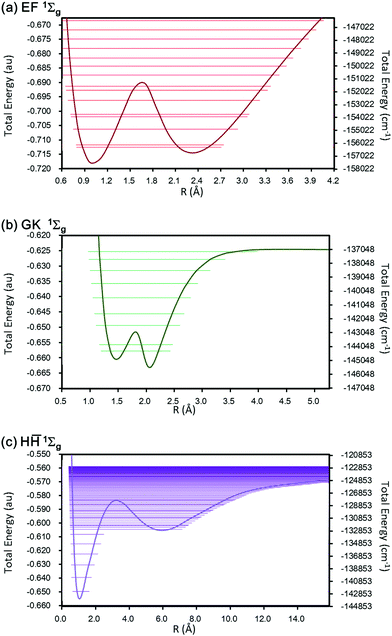Fig. 14 Vibrational energy levels (horizontal lines) associated with the double-well potential energy curves for the (a) EF, (b) GK, and (c) Hstates of the 1Σg symmetry.

For the EF state, the left- and right wells are similar to each other, but the right well is a little bit broad. The numbers of states assigned to the left well were 4 and 5 to the right. The left and right minima locate at R = 1.016 and 2.330 Å, respectively, and the position of the hill was 1.646 Å with the barriers: ΔELeft = 6290.14 cm−1 and ΔERight = 5572.46 cm−1. The v = 0 energy level lies below the v = 1 energy level by only 177.32 cm−1, and their 〈R〉 values lie at 1.047 Å for v = 0 and 2.353 Å for v = 1, as given in Table 3. We can clearly assign the v = 0 state to the left well (E state) and the v = 1 state to the right well (F state). The v = 2 level can be assigned to the right well since 〈R〉 = 2.413 Å. The v = 3 and v = 4 levels again lie close to each other with the splitting of only 182.45 cm−1, and they are assigned to the left and the right wells, respectively. The v = 5 level is assigned to the right well. The v = 6 energy level is calculated to be E = −152027.49 cm−1 = −0.692688 a.u., which is near the local maximum energy value of the E1 (EF) 1Σg potential curve, E = −0.690747 a.u. at R = 3.0 a.u., and the 〈R〉 value of the v = 6 state is 1.514 Å. This implies that the v = 6 state is delocalized over the two wells. The v = 7 level is somewhat localized again in the right well since the 〈R〉 values are about 2.305 Å. All of the vibrational states above v = 8 are delocalized and they are mixed states of E and F states since their absolute energies are higher than the top of the hill. If the double wells are completely symmetric, then two vibrational states should always become degenerate. In the present case, since the E and F shapes are similar to each other, the states: v = 0 and 1 and v = 3 and 4 nearly degenerate only with the energy gaps: 177.32 and 182.45 cm−1, respectively. Therefore, only with small thermal effects, the vibrational levels would shift between the left and the right by the tunneling process.

For the GK state, the energy barriers are quite small, i.e. ΔELeft = 1977.47 cm−1 and ΔERight = 2519.57 cm−1, compared with those of the EF and H. The number of the vibrational states below the hill, therefore, was only 2. The lowest one: v = 0 is assigned to the right well and the v = 1 state is assigned to the left well with the energy gap: 456.76 cm−1. Thus, each well has one vibrational state.

For the Hstate, the shape of the left well is sharp but that of the right side is very broad since it is on the ionic curve proportional to 1/R. A lot of vibrational states belonging to the right well were obtained. From v = 0 to 5, all their vibrational levels are assigned to the left well. The lowest vibrational state assigned to the right is v = 6. In the vibrational states of v = 0 to 27, the number of states belonging to the right well is 17 and, in contrast, only 4 to the left. The energy intervals between the neighboring two vibrational states belonging to the right well were calculated around 800 cm−1 (maximum) to 200 cm−1 (minimum). The energy gaps between v = 14 and 15 and v = 23 and 24 were incidentally small: 98.1 and 84.6 cm−1, respectively. However, the coupling of these vibrational states would be small because the positions of the left and right minima: R = 1.050 and 6.152 Å were apart and their energy barriers: ΔELeft = 15391.76 cm−1 and ΔERight = 5682.20 cm−1 were also very different from each other.

Similar discussions can be accomplished for the vibrational levels for the other double-well PECs as seen in the ESI. In the BO approximation, however, the vibrational analysis of a PEC having a complicated shape would highly depend on how to analyze the PEC, i.e. fitting function, the number of discrete points to fit the analytical curve, etc. Moreover, non-adiabatic effects become significant for these states. In such a case, we will need a non-BO treatment to obtain accurate vibrational states.11,12,46

## VI. Concluding remarks

We have systematically solved the Schrödinger equation of hydrogen molecules for the ground and excited states of the 1Σg, 1Σu, 3Σg, and 3Σu symmetries and obtained their accurate potential energy curves with the free complement variational method within the BO approximation. The calculated energies were very accurate, considered as essentially exact solutions, and guaranteed to be the upper bound to the exact energies due to the variational principle. We could describe the very small van der Waals minima (∼5 cm−1 depth) in the excited states very accurately. All the PECs were calculated in a common comprehensive manner according to the FC theory: the energies and wave functions of the ground and excited states were obtained simultaneously from the same secular equations. It guarantees that the wave functions of different states are orthogonal and Hamiltonian orthogonal to each other as shown by eqn (2) and (3). Therefore, even the complicated PECs that relate to the ionic contribution were also accurately described. To describe the dissociation limits accurately, it is better to introduce the ionic term and higher Rydberg functions corresponding to 2pz, 3pz, etc. orbitals of hydrogen atoms from the initial function. Since it may cause computational difficulty for integrations near dissociations, we did not introduce them to the present case. However, in the upcoming paper40 by the FC-LSE method where no integration problem occurs, these functions are introduced as the initial function and the PECs will be well described up to dissociation.

Based on the essentially-exact PECs by the FC theory, we also numerically solved the vibrational Schrödinger equations comprehensively for all the electronic ground and excited states calculated in this paper. In particular, we discussed the vibrational states on the double-well PECs of EF, GK, and Hstates of 1Σg. The double-well potential of the Hstate originates from the mixing of the ionic contributions. The present data and analysis should be valuable for experimental studies and useful as accurate reference data, in particular for future investigations in astronomical studies.

However, the non-adiabatic effects would often become significant especially for higher electronic excited states, and vibrational analysis often depends on computational conditions especially for the PECs having complicated or broad shapes. For these states, the non-BO approach is interesting11,12,46 and will be performed in the near future.

## Conflicts of interest

There are no conflicts to declare.

## Acknowledgements

This work was supported by the JSPS KAKENHI Grant Numbers 17KT0103, 17H06233, 26810014, 16K17864, and 16H02257. The computations were performed using the Research Center for Computational Science (IMS), Okazaki, Japan, and partially provided by the RIKEN Advanced Institute for Computational Science, Kobe, Japan, through the HPCI System Research Project (Project ID: hp140140). The authors sincerely thank the gracious support provided by these computer centers. The authors thank Dr T. Miyahara for valuable comments.

## References

1. H. Nakatsuji, J. Chem. Phys., 2000, 113, 2949 CrossRef CAS.
2. H. Nakatsuji, Phys. Rev. Lett., 2004, 93, 030403 CrossRef.
3. H. Nakatsuji, Phys. Rev. A: At., Mol., Opt. Phys., 2005, 72, 062110 CrossRef.
4. H. Nakatsuji, H. Nakashima, Y. Kurokawa and A. Ishikawa, Phys. Rev. Lett., 2007, 99, 240402 CrossRef CAS.
5. H. Nakatsuji, Acc. Chem. Res., 2012, 45, 1480 CrossRef CAS.
6. H. Nakashima and H. Nakatsuji, J. Chem. Phys., 2007, 127, 224104 CrossRef.
7. Y. I. Kurokawa, H. Nakashima and H. Nakatsuji, Phys. Chem. Chem. Phys., 2008, 10, 4486 RSC.
8. H. Nakashima and H. Nakatsuji, Phys. Rev. Lett., 2008, 101, 240406 CrossRef.
9. A. Ishikawa, H. Nakashima and H. Nakatsuji, J. Chem. Phys., 2008, 128, 124103 CrossRef.
10. A. Ishikawa, H. Nakashima and H. Nakatsuji, Chem. Phys., 2012, 401, 62 CrossRef CAS.
11. Y. Hijikata, H. Nakashima and H. Nakatsuji, J. Chem. Phys., 2009, 130, 024102 CrossRef.
12. H. Nakashima, Y. Hijikata and H. Nakatsuji, Astrophys. J., 2013, 770, 144 CrossRef.
13. Y. Kurokawa, H. Nakashima and H. Nakatsuji, Phys. Rev. A: At., Mol., Opt. Phys., 2005, 72, 062502 CrossRef.
14. A. Bande, H. Nakashima and H. Nakatsuji, Chem. Phys. Lett., 2010, 496, 347 CrossRef CAS.
15. H. Nakatsuji and H. Nakashima, TSUBAME e-Science J., 2014, 11(8), 24 Search PubMed.
16. H. Nakatsuji and H. Nakashima, J. Chem. Phys., 2015, 142, 084117 CrossRef.
17. H. Nakatsuji and H. Nakashima, J. Chem. Phys., 2015, 142, 194101 CrossRef PubMed.
18. H. M. James and A. S. Coolidge, J. Chem. Phys., 1933, 1, 825 CrossRef CAS; H. M. James and A. S. Coolidge, J. Chem. Phys., 1935, 3, 129 CrossRef.
19. J. S. Sims and S. A. Hagstrom, J. Chem. Phys., 2006, 124, 094101 CrossRef.
20. W. Kolos and L. Wolniewicz, J. Chem. Phys., 1965, 43, 2429 CrossRef CAS.
21. W. Kolos and L. Wolniewicz, Chem. Phys. Lett., 1974, 24, 457 CrossRef CAS.
22. L. Wolniewicz and K. Dressler, J. Chem. Phys., 1985, 82, 3292 CrossRef CAS.
23. L. Wolniewicz, J. Chem. Phys., 1993, 99, 1851 CrossRef CAS.
24. W. Kolos, J. Chem. Phys., 1994, 101, 1330 CrossRef CAS.
25. L. Wolniewicz and K. Dressler, J. Chem. Phys., 1994, 100, 444 CrossRef CAS.
26. T. Orlikowski, G. Staszewska and L. Wolniewicz, Mol. Phys., 1999, 96, 1445 CrossRef CAS.
27. G. Staszewska and L. Wolniewicz, J. Mol. Spectrosc., 1999, 198, 416 CrossRef CAS.
28. G. Staszewska and L. Wolniewicz, J. Mol. Spectrosc., 2002, 212, 208 CrossRef CAS.
29. See references in J. Rychlewski and J. Komasa, in Explicitly Correlated Wave Functions in Chemistry and Physics—Theory and Applications, ed. J. Rychlewski, Kluwer Academic, Dordrecht, 2003, pp. 91–147 Search PubMed.
30. W. Cencek, J. Komasa and J. Rychlewski, Chem. Phys. Lett., 1995, 246, 417 CrossRef CAS.
31. M. Stanke, D. Kdziera, S. Bubin, M. Molski and L. Adamowicz, J. Chem. Phys., 2008, 128, 114313 CrossRef.
32. G. Corongiu and E. Clementi, J. Chem. Phys., 2009, 131, 034301 CrossRef.
33. E. Clementi and G. Corongiu, Int. J. Quantum Chem., 2008, 108, 1758 CrossRef CAS.
34. K. Pachucki, Phys. Rev. A: At., Mol., Opt. Phys., 2010, 82, 032509 CrossRef.
35. K. Pachucki, Phys. Rev. A: At., Mol., Opt. Phys., 2013, 88, 022507 CrossRef.
36. J. W. Liu and S. Hagstrom, J. Phys. B: At., Mol. Opt. Phys., 1994, 27, L729 CrossRef CAS.
37. K. Pachucki and J. Komasa, Phys. Rev. A: At., Mol., Opt. Phys., 2011, 83, 042510 CrossRef.
38. T. E. Sharp, At. Data, 1971, 2, 119 CrossRef.
39. A. Pardo, Spectrochim. Acta, Part A, 1994, 50, 941 CrossRef.
40. H. Nakashima and H. Nakatsuji, J. Chem. Phys., submitted Search PubMed.
41. Computer code MAPLE, Waterloo Maple Inc., Waterloo, Ontario, Canada; see http://www.maplesoft.com.
42. About the GMP library, see http://www.cs.nyu.edu/exact/core/gmp.
43. R. J. Le Roy, Level 8.0: A Computer Program for Solving the Radial Schrödinger Equation for Bound and Quasibound Levels, University of Waterloo Chemical Physics Research Report CP-663, 2007; see http://leroy.uwaterloo.ca/programs/.
44. E. R. Davidson, J. Chem. Phys., 1960, 33, 1577 CrossRef CAS; E. R. Davidson, J. Chem. Phys., 1961, 35, 1189 CrossRef.
45. D. Bailly, E. J. Salumbides, M. Vervloet and W. Ubachs, Mol. Phys., 2010, 108, 827 CrossRef CAS.
46. H. Nakashima and H. Nakatsuji, J. Chem. Phys., 2013, 139, 074105 CrossRef.

### Footnote

Electronic supplementary information (ESI) available. See DOI: 10.1039/c8cp05949g
 This journal is © the Owner Societies 2019